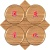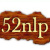0+0+0+0+0+0+0+0+0+0+0+0+0+0+0+0+0+0+0+0+0+Recently there has been an increase in the studies on time-series data mining specifically time-series clustering due to the vast existence of time-series in various domains. The large volume of data in the form of time-series makes it necessary to employ various techniques such as clustering to understand the data and to extract information and hidden patterns. In the field of clustering specifically, time-series clustering, the most important aspects are the similarity measure used and the algorithm employed to conduct the clustering. In this paper, a new similarity measure for time-series clustering is developed based on a combination of a simple representation of time-series, slope of each segment of time-series, Euclidean distance and the so-called dynamic time warping. It is proved in this paper that the proposed distance measure is metric and thus indexing can be applied. For the task of clustering, the Particle Swarm Optimization algorithm is employed. The proposed similarity measure is compared to three existing measures in terms of various criteria used for the evaluation of clustering algorithms. The results indicate that the proposed similarity measure outperforms the rest in almost every dataset used in this paper.0+0+0+下载预览0+0+0+0+0+0+0+0+0+0+0+0+0+0+0+0+0+0+0+0+0+
Top